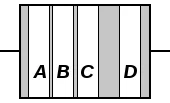# Resistor Color Code Calculator

The electronic color code is used to indicate the values or ratings of electronic components, usually for resistors, but also for capacitors, inductors, diodes and others. A separate code, the 25-pair color code, is used to identify wires in some telecommunications cables.

Standard Resistor and Capacitor values with IEC and EIA code is explained in previous post.

This tool is used to decode information for color banded axial lead resistors. Select their colors to determine the value and tolerance of the resistor.

### Understanding Color CodesTo distinguish left from right there is a gap between the C and D bands.

• band A is the first significant figure of component value (left side)
• band B is the second significant figure (some precision resistors have a third significant figure, and thus five bands).
• band C is the decimal multiplier
• band D if present, indicates tolerance of value in percent (no band means 20%)

For example, a resistor with bands of yellow, violet, red, and gold has first digit 4 (yellow in table below), second digit 7 (violet), followed by 2 (red) zeros: 4,700 ohms. Gold signifies that the tolerance is ±5%, so the real resistance could lie anywhere between 4,465 and 4,935 ohms.

## How does the resistor color code work?

Resistor values are often indicated with color codes. Practically all leaded resistors with a power rating up to one watt are marked with color bands. The coding is defined in the international standard IEC 60062. This standard describes the marking codes for resistors and capacitors. It includes also numerical codes, as for example often used for SMD resistors. The color code is given by several bands. Together they specify the resistance value, the tolerance and sometimes the reliability or failure rate. The number of bands varies from three till six. As a minimum, two bands indicate the resistance value and one band serves as multiplier. The resistance values are standardized, these values are called preferred value.

## Resistor color code chart

The chart below shows how to determine the resistance and tolerance for resistors. The table can also be used to specify the color of the bands when the values are known. An automatic resistor calculator can be used to quickly find the resistor values.Tight tolerance resistors may have three bands for significant figures rather than two, or an additional band indicating.